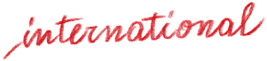# Pythagorean calculations

Tools and activities for learning the times tables easily

Product: Book

ISBN: 978-88-590-1814-8

Publication date: 01/03/2019

Suitable for: Primary 1st level (ages 6-7), Primary 2nd level (ages 8-10)

Learning the times tables means being able to have a set of facts, or results of simple operations already resolved, to be recalled immediately from the long-term memory or through a rapid calculation strategy, requiring the use of a known fact. As the child proceeds with arithmetic acquisitions, this competence develops in an increasingly complex way, but it is only with explicit teaching that an organized and flexible factual knowledge can be initiated. This volume – a completion of the book Numbers and Space, whose activities are focused on addition and subtraction calculation skills – uses a targeted strategy to create a significant learning program of multiplication and multiplication tables, which are an indispensable basis for the development of complex calculations. The illustrated activities and the attached tools have been designed to train and consolidate this learning, improving the motivation to learn, and the mastery and fluidity of calculations.

Contents: • Pythagorean times table chart and other tools • The learning program • Precursors  • Multiplication • The first times tables  • Squares  • Difficult times tables  • From multiplication to division Tools attached:  • Cards with multiple units • Personal times table chart  • Pythagorean times table chart • Cardboard “L” answer finder • Squares

Index

Introduction

Pythagorean times table chart

The learning program

Bibliography

Activities for learning the times tables

Thematic Areas